Next: Isothermal Atmosphere Up: Equilibrium of Compressible Fluids Previous: Equilibrium of Compressible Fluids

# Introduction

In this chapter, we investigate the equilibria of compressible fluids, such as gases. As is the case for an incompressible fluid (see Chapter 2), a compressible fluid in mechanical equilibrium must satisfy the force balance equation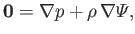(13.1)

whereis the static fluid pressure,the mass density, andthe gravitational potential energy per unit mass. In an ideal gas, the relationship betweenandis determined by the energy conservation equation, (1.89), which can be written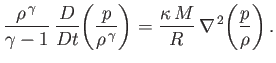(13.2)

Here,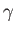is the ratio of specific heats,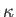the thermal conductivity,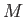the molar mass, and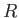the molar ideal gas constant. Note that the viscous heat generation term has been omitted from the previous equation (because it is zero in a stationary gas). The limits in which the left- and right-hand sides of Equation (13.2) are dominant are termed the adiabatic and isothermal limits, respectively. In the isothermal limit, in which thermal transport is comparatively large, so that Equation (13.2) can only be satisfied when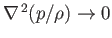, the temperature (recall that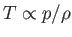in an ideal gas) distribution in the gas becomes uniform, and the pressure and density are consequently related according to the isothermal gas law,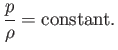(13.3)

On the other hand, in the adiabatic limit, in which thermal transport is negligible, so that Equation (13.2) can only be satisfied when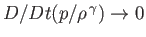, the pressure and density are related according to the adiabatic gas law,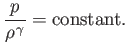(13.4)Next: Isothermal Atmosphere Up: Equilibrium of Compressible Fluids Previous: Equilibrium of Compressible Fluids
Richard Fitzpatrick 2016-03-31